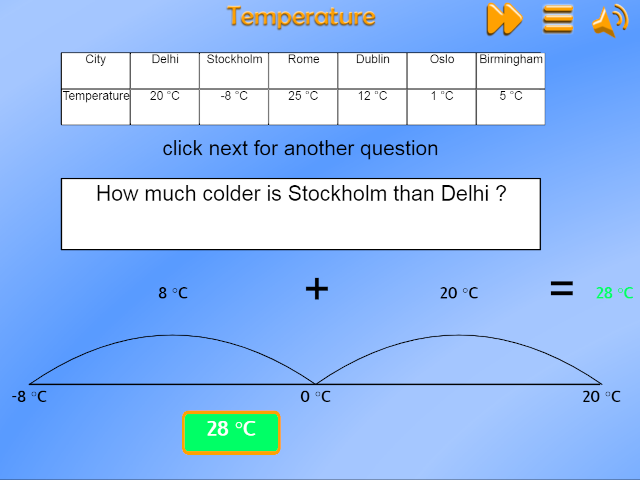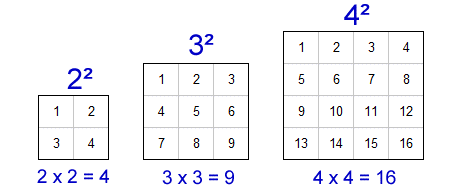# St. Patrick's Catholic Primary School

Making learning irresistible# Maths

## Place value

This term we are focusing on reading and writing numbers up to a million.

To understand number, we need to understand the value of each digit.

To do this we use a place value grid and children MUST remember these in order.

 Millions Hundred thousands Ten thousands Thousands Hundreds Tens Ones decimal point tenths hudredths M HTh TTh Th H T O . t h

## Roman numerals

In Year 4, children learnt Roman numerals to 100 (C).

This year we are learning Roman numerals to 1,000 (M).

Children MUST learn these by heart to work out the value.Remember:

1. The Romans were lazy and didn't repeat a letter more than 3 times e.g. III = 3, CCC = 300.
2. If a letter is placed after a letter that is worth more, you add the values because it is that many AFTER e.g. XV = 10 + 5 = 15.
3. If the letter is before a letter of higher value, you take it away because it is that many BEFORE                   e.g. IX = 1 before 10 so 10 - 1 = 9.  ONLY subtract I, X or C.

Rounding numbers

We have been learning this rhyme to help us round numbers to the nearest 10, 100 and 1,000.1. Circle the digit that the question asks you to round to nearest.
2. Look next door and decide if you need to add 1 more to the digit you circled, OR to let it rest and not change it.
3. Write the digits before (if there are any) down as they stay the same.
4. Write any digits after as 0's.

You can also check out this rap song

on YouTube at: Rounding numbers rap song

Comparing and ordering numbers

This video below (Ads at the start), will demonstrate how to use place value to compare and then order numbers by value.  Remember that some questions will ask you to order from smallest to largest or the greatest to the least.  Some questions may use the terminology ascending (going up) and descending (going down).  Read the question carefully!

Negative numbers

We use negative numbers in real life situations e.g. measuring cold temperatures or in an lift going to the basement floor of a department store.Any number below 0 is negative and any number above 0 is positive.

Positive numbers get higher the further we move right, so 5 is more than 2.

Negative numbers get lower the further we move left, so -5 is less than -2.

QUESTION

Which word, 'higher' or 'lower', would fit correctly in each of these gaps?

a) -7 is ............... than -1
b) + 62 is ............... than -71
c) -136 is ............... than -36

When finding the difference between a negative and positive number using a numberline, jump across each digit - don't forget that 0 is a number also.Due to Commutative law, it doesn't matter with addition (or in fact multiplication) the order in which the numbers are added (or multiplied) because you will get the same answer.  For example:

3 + 7 = 10 is the same as 7 + 3 = 10.

Column method:

1. You must align up the numbers correctly based on their value of each digit.  If you don't, you will get the wrong answer.

2. Always begin by adding up the ones column - because if you have more than 10 you need to exchange into the next column and you can't do this if you begin at the higher values.

3. Any column that have 10 or more, exchange for the next column and add 1 above the sum to include in the addition of that column.Bar models and whole-part models for addition:

We use models to visualise problems and to understand the calculation needed.

For the above example:

A bar model:A whole - part model:Remember that if you have a model (bar or whole-part) and the whole number is missing, then you MUST do addition of the parts.

Subtraction

Commutative law doesn't work with subtraction.  Subtraction law in primary schools, state that you must begin with the larger number first and take away from that (otherwise your answer will be negative numbers and we'll leave this for Secondary school, apart from easy calculations like 2 - 5 = -3).

Column method:

2. Begin with the ones column.  This is because you might need to exchange from higher values if you don't have enough to take away from.

3. If you need to, exchange from the next higher column and add convert into 10 lots of the column you are subtracting.  Don't forget to reduce the amount in the column you have subtracted from.  See the example below.

4. Remember that a number - 0 is the number.  Also, you can not take away from 0 so you will need to exchange from the next column.Bar models and whole-part models for subtraction:The question will have a part missing, to find it you must subtract from the whole the given part.

Whole - part models for subtraction

The model can be rotated on it's side like this example.  Just remember that the whole is the larger number.  To find the missing part, subtract the given part from the whole number.INVERSE

Some problems give you information and you need to do the inverse (OPPOSITE) calculation to workout the answer. For example:

Amy and Matthew are playing their favourite game. Amy's score is 8,524. Matthew's is bigger than Amy's and when you add them together their combined score is 19,384.
What is Matthew's score?

So we known from the question that  8,524 +  ?   = 19, 384

We need to do the inverse of addition to find the answer.

19,384 - 8,524 = 10, 860.

Matthew scored 10, 860.

Rounding for mental addition or subtraction

For quick calculations, particularly in shops when we don't have pen and paper handy, or in tests!!!!

1. Use the rounding rhyme to help round up or down the numbers in the calculations.

2. Complete the calculation for an approximate or estimation answer.

Question:

Mr Reid goes shopping  and buys milk £0.96, bread at £1.75 and Stilton cheese £3.53.

How much approximately has he spent?

Round up milk to £1.

Round down cheese to £3.50.

Cost approximately = £6.50.

Line graphs

Use line graphs to read data.

Remember to use the scales - x axis is horizontal along the bottom and the y axis is vertical going up and down.The y axis scale is increasing in 4 degrees C and the x axis shows the months.

Questions:

1. Which month had the biggest range in temperature? (biggest difference between maximum and minimum temperatures).
2. Which months had a maximum temperature of 16 degrees C?
3. What was the minimum overall temperature recorded? What month was this in?
4. How much warmer was Oct than Feb on the maximum temperature line?

Factors, multiples, primes and special numbers

Factors:

Factors are multiplied by each other e.g. 2  x 3  = 6.  The factors are 2 and 3 in this example.

1 is a factor of every number.

Multiples:

Multiples are the answers to a multiplication calculation, so in the example above, 6 is the multiple of 2 and 3.

0 is a multiple of every other number when they are multiplied by 0 e.g. 3 x 0 = 0,    120 x 0 = 0

1 is multiple of every number when it's multiplied by 1 e.g. 3 x 1 = 3, 120 x 1 = 120.

Question practice:

Which numbers is 24 is a multiple of ?

Work out which times tables 24 is in.   24 is a multiple of 1, 2, 3, 4, 6, 8, 12 and 24.

What are the factors of 24?  This is just another way of asking the same as the question above because the factors are 1, 2, 3, 4, 6, 8, 12 and 24.

Prime numbers:

These numbers are special as they only have 1 and it's own number as factors.  In other words, it can only be divided by 1 and it's own number.  It only appears in the 1 times table and it's own table.

We can rule out all even numbers except 2 (which is only a multiple of 1 and 2).  We can rule out all multiples of 5 except 5 (factors 1 and 5 only).  Prime numbers are odd except the number 2.

The prime numbers that pupils need to recall are:  2, 3, 5, 7, 11, 13, 17 and 19.

Square numbers:

Numbers that are multiplied by themselves can make a square multiplication array.  Therefore they are special numbers called SQUARE NUMBERS.Square numbers appear diagonally (red) in the multiplication grid.

Square numbers are written in this format:Cube numbers:

These special numbers are multiplied by themselves three times e.g. 3 x 3 x 3 = 27 because 3 x 3 = 9 which is then x 3 to make 27.

They are called cube numbers because they can make a 3D cube as a multiplication array.Cube numbers are written in this format:Games:

Numbergym